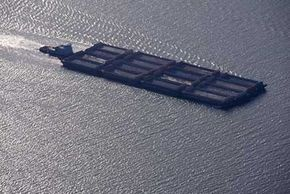# Why can boats made of steel float on water when a bar of steel sinks?A boat's average density is light compared to water's average density.
Stockbyte/Getty Images

The standard definition of floating was first recorded by Archimedes and goes something like this: An object in a fluid experiences an upward force equal to the weight of the fluid displaced by the object. So if a boat weighs 1,000 pounds (or kilograms), it will sink into the water until it has displaced 1,000 pounds (or kilograms) of water. Provided that the boat displaces 1,000 pounds of water before the whole thing is submerged, the boat floats.

It is not very hard to shape a boat in such a way that the weight of the boat has been displaced before the boat is completely underwater. The reason it is so easy is that a good portion of the interior of any boat is air (unlike a cube of steel, which is solid steel throughout). The average density of a boat -- the combination of the steel and the air -- is very light compared to the average density of water. So very little of the boat actually has to submerge into the water before it has displaced the weight of the boat.

The next question to ask involves floating itself. How do the water molecules know when 1,000 pounds of them have gotten out of the way? It turns out that the actual act of floating has to do with pressure rather than weight. If you take a column of water 1 inch square and 1 foot tall, it weighs about 0.44 pounds depending on the temperature of the water (if you take a column of water 1 cm square by 1 meter tall, it weights about 100 grams). That means that a 1-foot-high column of water exerts 0.44 pounds per square inch (psi). Similarly, a 1-meter-high column of water exerts 9,800 pascals (Pa).If you were to submerge a box with a pressure gauge attached (as shown in this picture) into water, then the pressure gauge would measure the pressure of the water at the submerged depth:

If you were to submerge the box 1 foot into the water, the gauge would read 0.44 psi (if you submerged it 1 meter, it would read 9,800 Pa). What this means is that the bottom of the box has an upward force being applied to it by that pressure. So if the box is 1 foot square and it is submerged 1 foot, the bottom of the box is being pushed up by a water pressure of (12 inches * 12 inches * 0.44 psi) 62 pounds (if the box is 1 meter square and submerged 1 meter deep, the upward force is 9,800 newtons). This just happens to exactly equal the weight of the cubic foot or cubic meter of water that is displaced!

It is this upward water pressure pushing on the bottom of the boat that is causing the boat to float. Each square inch (or square centimeter) of the boat that is underwater has water pressure pushing it upward, and this combined pressure floats the boat.